# CBSE Sample Papers for Class 12 Economics Paper 3

CBSE Sample Papers for Class 12 Economics Paper 3 are part of CBSE Sample Papers for Class 12 Economics. Here we have given CBSE Sample Papers for Class 12 Economics Paper 3.

## CBSE Sample Papers for Class 12 Economics Paper 3

 Board CBSE Class XII Subject Economics Sample Paper Set Paper 3 Category CBSE Sample Papers

Students who are going to appear for CBSE Class 12 Examinations are advised to practice the CBSE sample papers given here which is designed as per the latest Syllabus and marking scheme as prescribed by the CBSE is given here. Paper 3 of Solved CBSE Sample Paper for Class 12 Economics is given below with free PDF download solutions.

Time : 3 hrs
M.M.: 80

General Instructions

• All questions in both the sections A and B are compulsory. However, there is internal choice in questions of 3,4 and 6 marks.
• Question Nos. 1-4 and 13-16 are very short answer type questions, carrying 1 mark each.
They are required to be answered in one sentence each.
• Question Nos. 5-6 and 17-18 are short answer I type questions, carrying 3 marks each.
Answers to them should not normally exceed 60 words each.
• Question Nos. 7-9 and 19-21 are short answer II type questions, carrying 4 marks each.
Answers to them should not normally exceed 70 words each.
• Question Nos. 10-12 and 22-24 are long answer type questions, carrying 6 marks each.
Answers to them should not normally exceed 100 words each.
• Answers should be brief and to the point and the above word limit be adhered to as far as possible.

Section – A

Question 1.
Kamakshi’s brother Divij gave her ₹ 1,000 on the occassion of Rakshabandhan. She wants to buy a jeans which costs ₹ 900 and a pair of shoes which cost ₹ 600. However, with the . available amount, she cannot purchase both and has to choose amongst the two. Which problem is being faced by Kamakshi?

Question 2.
When there are diminishing returns to factor, total product first increases at increasing rate and then starts falling. The given statement is
(a) True
(b) False
(c) Partly True
(d) None of these

Question 3.
Draw fixed cost curve.

Question 4.
For a firm, Total Cost= Total Profit= ₹ 100. This is a situation of
(a) abnormal profit
(b) normal profit
(c) break-even point
(d) Both (b) and (c)

Question 5.
Vishnu consumes two commodities X and Y, whose prices are ₹ 16 and ₹ 24 per unit whether Vishnu is in equilibrium? If not, then tell what should he do to achieve equilibrium.
Or
Differentiate between individual demand curve and market demand curve.

Question 6.
Gulf countries have acquired more capital goods by selling oil to various countries. What would be its impact on Production Possibility Curve?

Question 7.
Explain monopoly and monopolistic competition.

Question 8.
Price of a commodity rises from ₹ 5 to ₹ 6. As a result, its demand falls from 100 units to 80 units. Find out Price Elasticity of Demand (Ed) by percentage method.
Or
If a fact is given that the slope of a straight line demand curve = $$\frac { \triangle }{ \triangle Q }$$, how do you relate it to own price elasticity of demand, Also, find price elasticity of demand, if demand is perfectly elastic.

Question 9.
A firm’s fixed cost is ₹2,000. Compute Total Variable Cost (TVC), Average Variable Cost (AVC), Total Cost (TC) and Average Cost (AC) with the help of the following table.

 Output (units) 1 2 3 4 5 6 7 Marginal Cost (MC) (₹) 2,000 1,500 1,200 1,500 2,000 2,700 3,500

Question 10.
Explain with the help of diagram, the effect of following changes on the demand for a commodity.
(i) A fall in the price of complementary goods
(ii) A rise in the price of substitute goods.

Question 11.
State the Law of Supply, the assumptions on which it is based and exceptions to the law of supply.

Question 12.
When both demand and supply fall simultaneously, what will be the effect on
Or
Explain the effect of decrease in supply when
(i) demand is perfectly elastic
(ii) demand is perfectly inelastic

Section – B

Question 13.
What do you mean by Escheat?

Question 14.
Interest received on loans is a capital receipt of the government. Do you agree?

Question 15.
In case of credit money
(a) Money value = Commodity value
(b) Money value > Commodity value
(c) Money value < Commodity value
(d) None of these

Question 16.
What type of losses a depositor has to bear if he withdraws his fixed deposit before the due date of maturity?

Question 17.
In an economy, investment increases by ₹ 100 crore. As a result, income increases by ₹ 250 crore. What is the value of multiplier? What does this imply?

Question 18.
Distinguish between voluntary unemployment and involuntary unemployment.
Or
Write any three points of difference between ex-ante investment and ex-post investment.

Question 19.
Find compensation of employees.

 S.No. Items (₹) in crores (i) Profit 500 (ii) Net Domestic Product at Factor Cost (NDPFC) 4,000 (iii) Interest 400 (iv) Depreciation in the Value of Fixed Capital 800 (v) Mixed Income 1,500 (vi) Indirect Tax 300 (vii) Subsidies 100 (viii) Rent 600

Question 20.
In a developing economy, Central Bank plays a prominent role. Comment.
Or
Briefly explain the components of money supply in India.

Question 21.
‘Mission Develop India’ is the plan of government to create basic infrastructure. It comprises of projects on irrigation, roads, housing, water supply and telecommunication connectivity. Above stated example is a government expenditure or government revenue. Elaborate it. Also, state the impact of this programme on rural growth.

Question 22.
Calculate Gross Domestic Product at Market Price ( ) by using
(i) Product Method
(ii) Income Method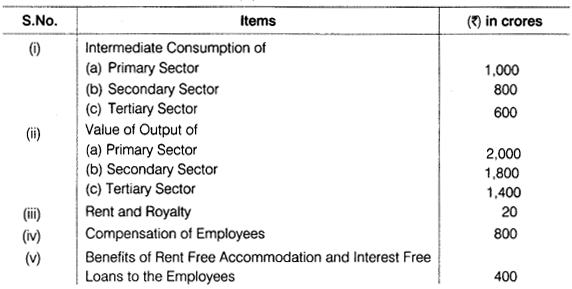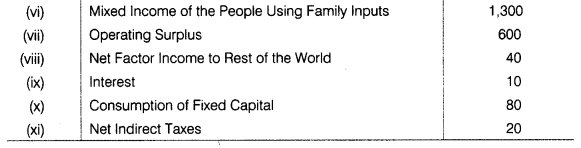Question 23.
What do you understand by Aggregate Demand (AD)? Also explain its components briefly.
Or
Given that National Income is ₹ 80 crore and Consumption Expenditure is ₹ 64 crore, find out Average Propensity to Save (APS). When income rises to ₹ 100 crore and Consumption Expenditure to 178 crore, what will be the Average Propensity to Consume (APC) and Marginal Propensity to Consume (MPC)?

Question 24.
How is exchange rate determined under flexible exchange rate system? Also enumerate the sources of demand and supply of foreign exchange. If demand for foreign exchange is continuously rising then which economic value stands affected?

Kamakshi is facing problem of choice. This problem arises because wants are unlimited and resources are scarce and have alternative uses.

(b) The given statement is false.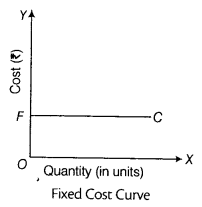(d) Both (b) and (c)

A consumer strikes his equilibrium when, $$\frac { M{ U }_{ X } }{ { P }_{ X } } =\frac { M{ U }_{ Y } }{ { P }_{ Y } }$$
Now, as per the given information, $$\frac { M{ U }_{ X } }{ { P }_{ X } } =\frac { 6 }{ 16 } =\frac { 3 }{ 8 }$$ and $$\frac { M{ U }_{ Y } }{ { P }_{ Y } } =\frac { 4 }{ 24 } =\frac { 1 }{ 6 }$$
∴ $$\frac { M{ U }_{ X } }{ { P }_{ X } } \neq \frac { M{ U }_{ Y } }{ { P }_{ Y } }$$
So, Vishnu is not in equilibrium
Now, $$\frac { M{ U }_{ X } }{ { P }_{ X } } >\frac { M{ U }_{ Y } }{ { P }_{ Y } } \left[ \because \frac { 3 }{ 8 } >\frac { 1 }{ 6 } \right]$$
Therefore, to attain equilibrium, Vishnu should start consuming more of Good X and less of Good Y so that
MU of good X declines and MU of Good Y increases till the point. Where $$\frac { M{ U }_{ X } }{ { P }_{ X } } =\frac { M{ U }_{ Y } }{ { P }_{ Y } }$$
Or
Difference between individual demand curve and market demand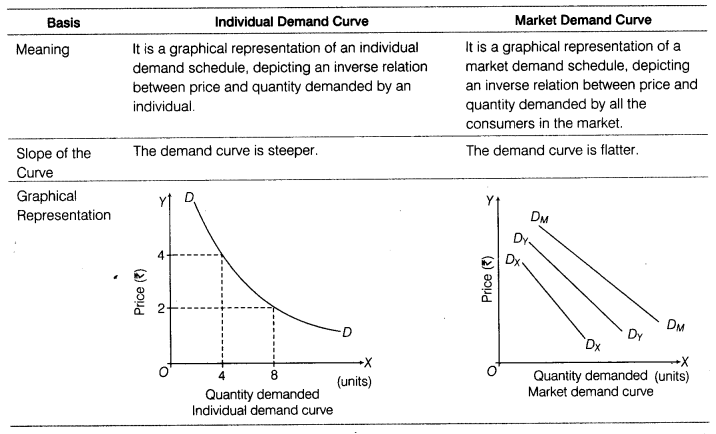It is an example of growth of resources. Therefore, it results in rightward shift of Production Possibility Curve. It leads to higher level of output for the gulf countries.
It is depicted in the graph given below :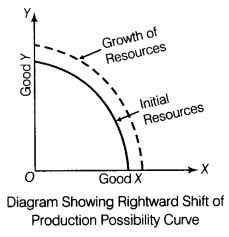Monopoly It refers to that form of market in which there is a single firm (or producer) producing a good/service, for which there are no close substitutes, e.g. Indian Railways, Reserve Bank of India, etc.
The main features of monopoly are stated below :

1. Single producer
2. No close substitutes
3. Barriers to entry
4. Independent price policy
5. Price discrimination is possible

Monopolistic Competition It is a market situation in which there are many sellers of a particular product but the product of each seller is differentiated from the other, e.g. soaps and toothpastes.
The main features of monopolistic competition are stated below :

1. Large number of buyers and sellers
2. Freedom of entry or exit
3. Product differentiation
4. Imperfect knowledge
5. Selling costs
6. High transportation costs

Here, price and quantity for the commodity are as follows:

 Price (₹) Quantity (Units) 5 100 6 80

Given,
Initial Price (P)= ₹ 5 Change in Price(∆P) = ₹ 1(6-5)
Initial Quantity (Q) = 100 units Change in Quantity (∆Q) = 20 units (100 – 80)
We know that,
Elasticity of Demand $$\left( { E }_{ d } \right) =\left( – \right) \frac { \triangle Q }{ \triangle P } \times \frac { P }{ Q }$$
$$=\left( – \right) \frac { 21 }{ 1 } \times \frac { 5 }{ 100 }$$
∴ Ed = (-1)
Or
We know that, price elasticity of demand $$\left( { E }_{ d } \right) =-\frac { \triangle Q }{ \triangle P } \times \frac { P }{ Q }$$
It is given that, the slope of demand curve = $$\frac { \triangle P }{ \triangle Q }$$
We can write that, $${ E }_{ d }=\frac { 1 }{ Slope\quad of\quad Demand\quad Curve } \times \frac { P }{ Q }$$
Where, P = Initial Price, Q = Initial Quantity, ∆P = Change in Price ∆Q = Change in Quantity
So it follows that, price elasticity of demand is the reciprocal of the slope of the demand curve multiplied by the ratio of price to quantity.
If demand is perfectly elastic, slope of demand cunve = 0
By formula,
$${ E }_{ d }=\frac { 1 }{ Slope\quad of\quad Demand\quad Curve } \times \frac { P }{ Q } =\frac { 1 }{ 0 } \times \frac { P }{ Q } =\infty$$
∴ Price elasticity of demand is at infinity, if demand is perfectly elastic.

Cost Schedule

 Output (Q) MC TFC TVC TC AVC AC 1 2,000 2,000 2,000 4,000 2,000 4,000 2 1,500 2,000 3,500 5,500 1,750 2,750 3 1,200 2,000 4,700 6,700 1,567 2,233 4 1,500 2,000 6,200 8,200 1,550 2,050 5 2,000 2,000 8,200 10,200 1,640 2,040 6 2,700 2,000 10,900 12,900 1,817 2,150 7 3,500 2,000 14,400 16,400 2,057 2,343

Formulae used
TVC = ΣMC
AVC = TVC / units of output
TC = TFC + TVC
AC = TC / units of output

(i) Complementary Goods It refers to those goods, which are used together for satisfaction of wants, e.g., car and petrol, pen and refill, needle and thread, tea and sugar, etc.
Effect of Fall in the Price of Complementary Goods The demand for a commodity increases with the fall in the price of complementary goods. If there is a fall in the prices of complementary goods, the demand curve shifts to the right which shows increase in demand at a given price of the commodity itself. The shifting of demand curve for a commodity to the right with fall in the price of complementary good is shown in the diagram given below :
Diagram Showing Increase in Demand for Complementary Goods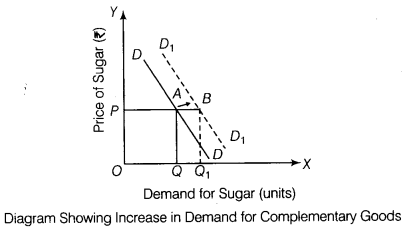(ii) Substitute Goods
These are those goods which can be substituted for each other, such as tea and coffee, ball pen and ink pen, etc.
Effect of Rise in the Price of Substitute Goods In case of such goods, increase in the price of one causes increase in the demand for the other, e.g. demand curve of tea shifts to right when price of the substitute commodity, i.e. coffee increases.
The shifting of demand curve for a commodity to the right with rise in the price of substitute good is shown in the diagram given below :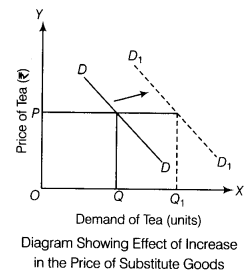Law of supply derives the relationship between price and quantity supplied. According to this law, quantity supplied of a commodity is directly related to the price of a commodity, provided all other determinants are constant. The quantity supplied increases with increase in price and vice-versa.
Assumptions of the Law of Supply
The various assumptions of the Law of Supply are given below :

1. There is no change in the price of the factors of production.
2. There is no change in the techniques of production.
3. There is no change in the goal of the firm.
4. There is no change in the price of related goods.

Exceptions to the Law of Supply
The following are the exceptions to the Law of Supply :

1. Agricultural Goods Law of Supply does not apply for agricultural goods, as their supply depends on climatic conditions and not on price.
2. Perishable Goods Perishable goods like fruits, vegetables, milk and milk products cannot be held for long. Therefore, suppliers are willing to supply these products, even when prices are less, for fear that they would become totally useless.
3. Antique Goods, Rare Articles and Paintings These goods are highly priced, but still their supply is limited, as supply here is affected by factors other than price, e.g., the supply of Hussain’s paintings cannot be increased even if buyers are willing to pay high price for it.
4. Future Expectations Regarding Prices If prices are rising, but sellers anticipate that they would rise further in future, then they would not increase their supply now.
5. Lack of Resources In underdeveloped or backward economies, supply cannot be increased due to lack of resources.
6. Labour Market In the labour market, it is observed that as the wage rate rises, the workers tend to work for less hours, so as to enjoy more leisure. This causes the supply of workers to decrease at increased wage rate.

Equilibrium Price The price at which market demand equals market supply is termed as equilibrium price: When demand and supply both fall simultaneously, there are three possible conditions under this situation :
(i) No Change in Equilibrium Price If there is proportionately equal change in demand and supply, the equilibrium price will remain constant, as shown in the following figure :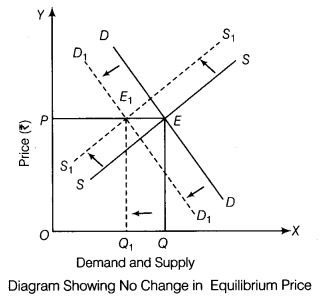(ii) Equilibrium Price can Fall
If decrease in demand is greater than decrease in supply, then equilibrium price will fall, as shown in the following figure :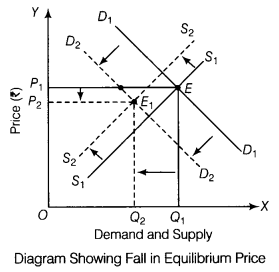(iii) Equilibrium Price can Rise
If decrease in demand is less than decrease in supply, then equilibrium price will increase, as shown in the following figure :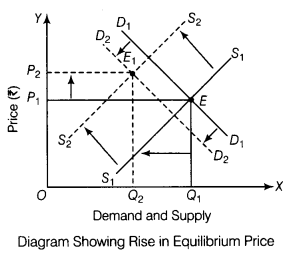The effect of decrease in supply when

(i) Demand is Perfectly Elastic Decrease in supply of commodity does not cause any change in its price in case demand for the commodity is perfectly elastic. Only equilibrium quantity tends to change.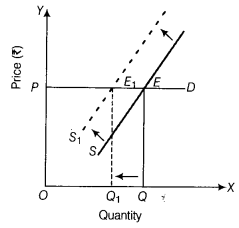Demand is infinite at existing price. So, decrease in supply does not cause any change in price.
(ii) Demand is Perfectly Inelastic Decrease in supply of commodity causes rise in price and equilibrium quantity remains constant when demand for commodity is inelastic.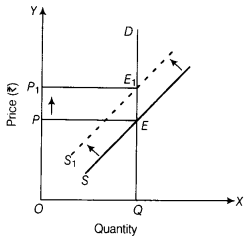Demand is constant at every price. So, decrease in supply does not cause any change in price.

Section – B

Escheat is the revenue which government makes out of the property that people leave without a legal heir.

No, this is a revenue receipt of the government, as it neither increase liabilitie nor reduce assets.

(b) Money value > Commodity value

A depositor will loose interest and certain amount out of the principal money, if he withdraws his fixed . deposits before due date. It is a financial loss.

Given, Increase in Investment (∆/) = ₹ 100 crore; Increase in Income (∆Y) = ₹ 250 crore
We know that, Investment Multiplier $$\left( K \right) =\frac { \triangle Y }{ \triangle l } \Rightarrow \frac { \triangle Y }{ \triangle l } =\frac { 250 }{ 100 } =2.5$$
This implies that for every unit increase in investment, income increases 2, 5 times.

Difference between voluntary unemployment and involuntary unemployment are :

 Basis Voluntary Unemployment Involuntary Unemployment Meaning It is a situation when people are offered work but they are not willing to work. It is a situation when people are willing to work but they are not getting work. Reason It occurs because of low wages or substandard jobs being offered. It occurs due to lack of employment opportunities. Nature It is avoidable. It is unavoidable.

Or
Difference between ex-ante Investment and ex-post Investment are

 Basis Ex-ante Investment Ex-post Investment Meaning Ex-ante investment refers to the planned or desired level of investment. Ex-post investment refers to the actual or realised level of investment. Relation with savings In an accounting year, ex-ante investment may or may not be equal to ex-ante savings In an accounting year, ex-post investment is always equal to actual savings (in accordance with national income accounting principle). Relevance in determining Equilibrium level of income is determined where ex-ante investment = ex-ante saving. Ex-post investment has no relevance in determination of equilibrium level of income.

Net Domestic Product at Factor Cost (NDPFC)
NDPFC Compensation of Employees + Operating Surplus (Profit + Interest-)- Rent) + Mixed Income
∴ Compensation of Employees = NDPFC – Operating Surplus – Mixed Income
= 4,000 – (500 + 400 + 600) – 1,500
= 4,000 – 3,000 = ₹ 1,000 crore
So, compensation of employees = ₹ 1,000 crore

In a developing economy like India, the main objective of central banking is to promote economic growth.
The role of a Central Bank in a developing economy is discussed below :

1. Control and Regulation of Banking System The Central Bank is expected to perform its traditional functions of controlling and directing the banking structure of the country.
2. Promotional Functions Since the monetary and banking structure in a developing economy is backward, the Central Bank helps in the expansion and promotion of the banking structure.
3. Developmental Functions The main problem of developing economies is economic development. The Central Bank must, therefore, take all those steps which help in the advancement of economic growth.

Or
Money supply refers to the stock of money in circulation in an economy at a given point of time. Money supply includes currency in the form of paper money and coins held by individual and business firms and net demand deposits held by commercial banks.
According to M, money measure, money supply has following components:
M1 = C + DD + OD
Here, C is the currency held by public, DD refers to demand deposit of people with commercial bank and OD includes demand deposits with RBI of other institutions and government.

It is an example of government expenditure, the principle component of fiscal policy. Such expenditure of government on construction of roads, education, defence of the country are made by the government to correct the situation of excess demand or deficient demand in the economy.
When there is excess demand, government expenditure is reduced and when there is a deficient demand, government expenditure is increased.
It is a flagship programme by the government of India. This project is an investment in rural infrastructure which will unlock the growth potential of rural India.

(i) By Product Method
Gross Domestic Product at Market Price (GDPmp)
=Value of Output (Primary Sector +Secondary Sector + Tertiary Sector)
– Intermediate Consumption (Primary Sector + Secondary Sector + Tertiary Sector)
= (2,000 + 1,800 + 1,400) – (1,000 + 800 + 600) = 5200 – 2,400
GDPMP = ₹ 2,800 crore

(ii) By Income Method
Net Domestic Product at Factor Cost (NDPFC)
= Compensation of Employees + Operating Surplus + Mixed Income
= 800 + 600 + 1,300 = ₹ 2,700 crores
GDPMP = NDPFC + Net Indirect Tax + Depreciation
= 2,700 + 20 + 80 = ₹ 2,800 crore

Aggregate Demand (AD) refers to the sum total of expenditure that the people plan to incur on the purchase of goods and services produced in an economy (during the period of an accounting year) corresponding to their different levels of income.
Components of AD are: AD = C + I + G + (X-M)

1. Household Consumption Expenditure (C) This measures the demand for consumer goods .i.e. that expenditure which people wish to make on consumer goods corresponding to different levels of income in economy.
2. Producer Investment Expenditure (/) This measures the demand for producer goods which lead to capital formation i.e. that expenditure which people wish to make on producer goods corresponding to different levels of income in economy.
3. Government Expenditure (G) It refers to public consumption expenditure (such as purchase of foods and clothes for military personnel) and investment expenditure (such as construction of road) made by government.
4. Net Exports (X – M) Exports (X) increase aggregate demand in domestic economy, while imports (M) decrease it. Therefore, value of net exports (X – M) contributes to AD in domestic economy.

Or
Here in first condition, Y = ₹ 80 crore and C = ₹ 64crore
Hence, S = Y – C = 80 – 64 = ₹ 16crore
Now, Average Propensity to Save (APS)
$$APS=\frac { S }{ Y } =\frac { 16 }{ 80 } =0.20$$
Again, when income and consumption expenditure rise,
Y = ₹ 100 crore and C = ₹ 78 crore
So, Average Propensity to Consume (APC)
$$APS=\frac { C }{ Y } =\frac { 78 }{ 100 } =0.78$$
Here, ∆Y = 100 – 80 = ₹ 20 crore
∆C = 78 – 64 = ₹ 14 crore
So, Marginal Propensity to Consume (MPC)
$$MPC=\frac { \triangle C }{ \triangle Y } =\frac { 14 }{ 20 } =0.70$$
Where, ∆C = Change in Consumption, ∆Y = Change in Income
∆/ = Change in Investment, K = Multiplier
MPC = Marginal Propensity to Consume, APC = Average Propensity to Consume
APS = Average Propensity to Save

Foreign exchange rate is determined by the demand and supply of foreign exchange.
The rate of foreign exchange is said to be in equilibrium, when the demand for some currency in terms of another currency equals its supply.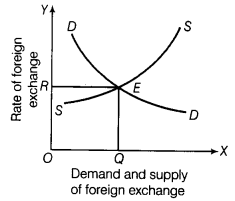In the given figure, the line SS shows the supply of foreign currency which is positively related to the rate of exchange. The line DD shows the demand for foreign currency which is negatively related to the rate of exchange. The point £, at which demand is equal to supply, represents the equilibrium rate of exchange.
Sources of Demand for Foreign Exchange
Foreign exchange is demanded for the following purposes :

1. Purchase of foreign goods by domestic residents, i.e. imports.
2. Payments of international loans.
3. Gifts and grants to the rest of the world.
4. Investments in the rest of the world.